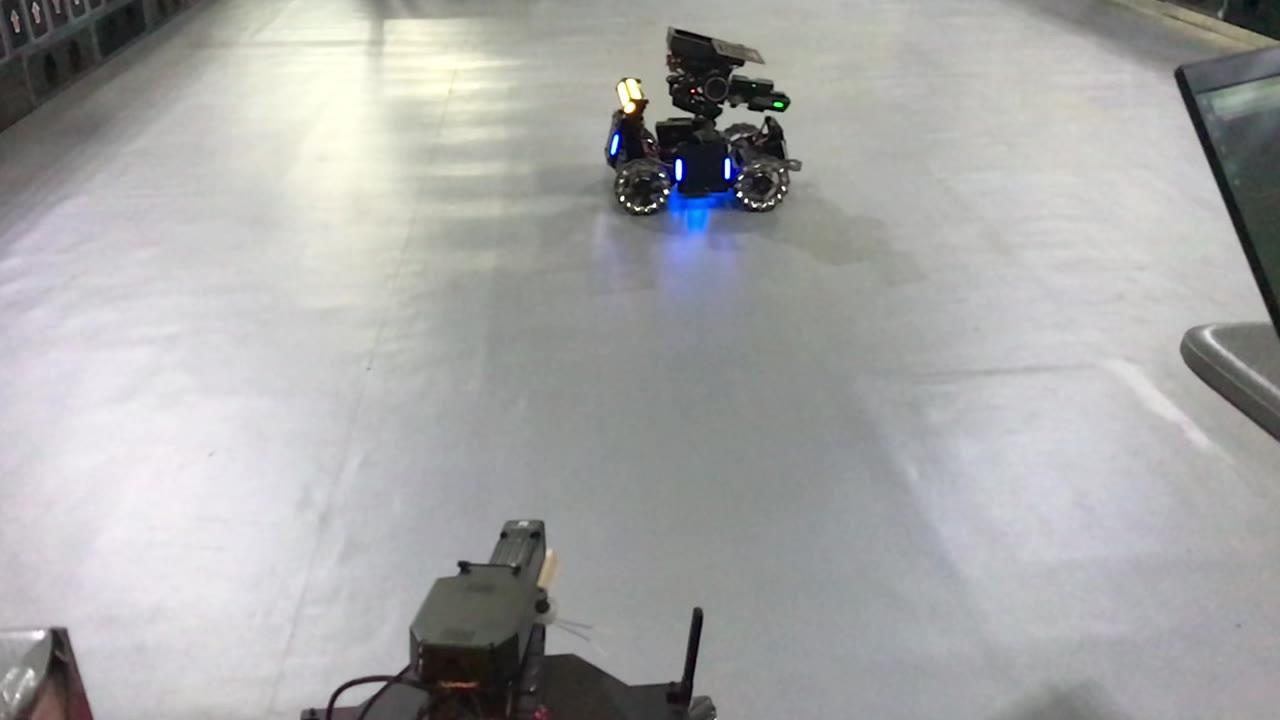## 2.1 插值+低通滤波

MATLAB仿真结果如下（截止频率为10Hz），延迟大概有150ms：

## 3. 卡尔曼滤波器介绍

《An Introduction to the Kalman Filter》

Understanding Kalman Filters

## 3.3.1 设定H是状态变量到测量值的转换矩阵

w和v分别是过程噪声和测量噪声，假设两者相互独立，且都是正态分布的白噪声（高斯白噪声），满足以下条件：• Q、R分别是过程噪声和测量噪声的协方差矩阵（对角线上是方差，其他是元素的协方差），假设它们是定值。R可以根据传感器数据大概估算出来，Q是依赖模型的准确度，需要调试。
•先验状态估计（预测值）
•后验状态估计（估计值）
•先验误差协方差
•后验误差协方差

## 3.3.2 递推过程

1. 计算预测值、预测值和真实值之间的误差协方差矩阵2. 计算卡尔曼增益，得到估计值3. 计算估计值和真实值之间误差协方差矩阵，为下次递推做准备经过状态转化方程得到估计值是测量值，根据两者的置信度得到最优估计值3.3.3 参数对估计效果的影响

A、B、H根据模型来确定。决定了滤波器的稳态频率响应，Q↑，R↓，都会导致卡尔曼增益K变大，从而使得估计值倾向于测量值（包含更多的高频噪声信号）

P变大也会导致卡尔曼增益变大，但是很快会迭代至稳定值，因此P的初始值只会对前几个周期的估计值有影响。

## 3.3.4 卡尔曼滤波与互补滤波的关系## 1.（一维）卡尔曼滤波器## 4.2 坐标系和运动学模型的选择

• 恒定速度模型（Constant Velocity, CV）
• 恒定加速度模型（Constant Acceleration, CA）

• 恒定转率和速度模型（Constant Turn Rate and Velocity，CTRV）
• 恒定转率和加速度模型（Constant Turn Rate and Acceleration，CTRA）

## 1.观测量选择为x,y坐标x方向滤波结果如下，图上方的两条是位置曲线，下方是速度曲线

x方向结果如下

## 4.5 相机转移到云台上https://www.zhihu.com/video/996642206844821504https://www.zhihu.com/video/996651228453101568

## 5. 卡尔曼滤波器在STM32平台的实现和MATLAB仿真代码

1. 调用开源的矩阵运算库，效率较低
2. 将矩阵运算拆开成基本的四则运算，扩展性很差，例如改变矩阵阶数就没办法用了，而且在遇到例如求逆运算的时候比较麻烦
3. 调用STM32自带的DSP库中的矩阵运算功能，可以根据需求自行添加，简单高效。

/* second-order kalman filter on stm32 */
#include "stm32f4xx_hal.h"
#include "arm_math.h"

#define mat         arm_matrix_instance_f32
#define mat_init    arm_mat_init_f32
#define mat_sub     arm_mat_sub_f32
#define mat_mult    arm_mat_mult_f32
#define mat_trans   arm_mat_trans_f32
#define mat_inv     arm_mat_inverse_f32

typedef struct
{
float raw_value;
float filtered_value;
mat xhat, xhatminus, z, A, H, AT, HT, Q, R, P, Pminus, K;
} kalman_filter_t;

typedef struct
{
float raw_value;
float filtered_value;
float xhat_data, xhatminus_data, z_data,Pminus_data, K_data;
float P_data;
float AT_data, HT_data;
float A_data;
float H_data;
float Q_data;
float R_data;
} kalman_filter_init_t;

void kalman_filter_init(kalman_filter_t *F, kalman_filter_init_t *I)
{
mat_init(&F->xhat,2,1,(float *)I->xhat_data);
...
mat_init(&F->HT,2,2,(float *)I->HT_data);
mat_trans(&F->H, &F->HT);
}

float *kalman_filter_calc(kalman_filter_t *F, float signal1, float signal2)
{
float TEMP_data = {0, 0, 0, 0};
float TEMP_data21 = {0, 0};
mat TEMP,TEMP21;

mat_init(&TEMP,2,2,(float *)TEMP_data);
mat_init(&TEMP21,2,1,(float *)TEMP_data21);

F->z.pData = signal1;
F->z.pData = signal2;

//1. xhat'(k)= A xhat(k-1)
mat_mult(&F->A, &F->xhat, &F->xhatminus);

//2. P'(k) = A P(k-1) AT + Q
mat_mult(&F->A, &F->P, &F->Pminus);
mat_mult(&F->Pminus, &F->AT, &TEMP);

//3. K(k) = P'(k) HT / (H P'(k) HT + R)
mat_mult(&F->H, &F->Pminus, &F->K);
mat_mult(&F->K, &F->HT, &TEMP);

mat_inv(&F->K, &F->P);
mat_mult(&F->Pminus, &F->HT, &TEMP);
mat_mult(&TEMP, &F->P, &F->K);

//4. xhat(k) = xhat'(k) + K(k) (z(k) - H xhat'(k))
mat_mult(&F->H, &F->xhatminus, &TEMP21);
mat_sub(&F->z, &TEMP21, &F->xhat);
mat_mult(&F->K, &F->xhat, &TEMP21);

//5. P(k) = (1-K(k)H)P'(k)
mat_mult(&F->K, &F->H, &F->P);
mat_sub(&F->Q, &F->P, &TEMP);
mat_mult(&TEMP, &F->Pminus, &F->P);

F->filtered_value = F->xhat.pData;
F->filtered_value = F->xhat.pData;

return F->filtered_value;
}

%% 一阶卡尔曼滤波与低通滤波器对比
% 初始化参数
ts = 0.001;                 % 采样时间
delta_t  = 0.02;            % 实际信号帧率
noise_mag = 1;              % 设置噪声幅度为1
t = 0:ts:2-ts;
t2 = 0:delta_t:2-delta_t;
N = length(t);              % 序列的长度
sz = [1,N];                 % 信号需开辟的内存空间大小
x = 20*sin(2*pi*t);         % 真实位置
noise = noise_mag*randn(1,N*ts/delta_t);              % 测量白噪声
z = interp1 (t2,20*sin(2*pi*t2)+noise,t,'previous');  % 信号叠加噪声后重新采样
Q = 1;                      % 假设建立的模型噪声方差
R = 2000;                   % 位置测量方差估计

A=1;
B=0;
H=1;

n=size(Q);
m=size(R);

% 分配空间
xhat=zeros(sz);         % 后验估计
P=0;                    % 后验方差估计
xhatminus=zeros(sz);    % 先验估计
Pminus=zeros(n);        % 先验方差估计
K=zeros(n(1),m(1));     % Kalman增益
I=eye(n);

% 估计的初始值都为默认的0，即P=[0 0;0 0],xhat=0
for k = 2:N
% 时间更新过程
xhatminus(:,k) = A*xhat(:,k-1)+B;
Pminus= A*P*A'+Q;

% 测量更新过程
K = Pminus*H'/( H*Pminus*H'+R );
xhat(:,k) = xhatminus(:,k)+K*(z(k)-H*xhatminus(:,k));
P = (I-K*H)*Pminus;
end
F = filter_custom;
z_lp = filter(F,z);
x_k = cat(1,x(1:1950),xhat(1:1950));
distance_k = pdist(x_k); % 计算两个向量的欧氏距离作为偏差的判定
x_lp = cat(1,x(1:1950),z_lp(1:1950));
distance_lp = pdist(x_lp);
x_z = cat(1,x(1:1950),z(1:1950));
distance_z = pdist(x_z);

figure;
plot(t,x(1,:),'g-');
hold on
plot(t,z);
plot(t,xhat(1,:),'r-')
plot(t,z_lp,'k-');
legend('truth',['measurement ',num2str(distance_z)], ['kalman filter ',num2str(distance_k)], ['lowpass ',num2str(distance_lp)]);
xlabel('time(s)');

function Hd = filter_custom
% Chebyshev Type II Lowpass filter designed using FDESIGN.LOWPASS.

% All frequency values are in Hz.
Fs = 1000;  % Sampling Frequency

N     = 10;  % Order
Fstop = 20;  % Stopband Frequency
Astop = 80;  % Stopband Attenuation (dB)

% Construct an FDESIGN object and call its CHEBY2 method.
h  = fdesign.lowpass('N,Fst,Ast', N, Fstop, Astop, Fs);
Hd = design(h, 'cheby2');
end